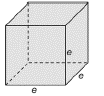Chapter 9.1, Problem 32EElementary Geometry For College St...

7th Edition
Alexander + 2 others
ISBN: 9781337614085

Solutions

Chapter
SectionElementary Geometry For College St...

7th Edition
Alexander + 2 others
ISBN: 9781337614085
Textbook Problem

Use the formulas and drawing in Exercise 31 to find ( a ) the total area T and (b) the volume V of a cube with edges of length 5.3 ft each.A cube is a right square prism in which all edges have the same length. For the cube with edge e , a ) Show that the total area is T = 6 e 2 . b ) find the total area if e = 4 cm. c ) Show that the volume is V = e 3 . d ) find the volume if e = 4 c m .To determine

a)

The total area T and.

Explanation

The total area of any solid is the area of all the number of lateral sides that it have, including the area of the top and the bottom of solid.

The volume of any solid is the region of space occupied by it and in general the volume of a solid is given by the product of its base area and altitude.

Calculation:

Given,

The cube of edge e=5.3 ft.

From exercise 31, the total area T of the cube is given by

T=6e2

But, we have e=5

To determine

b)

The volume V of a cube with edges of length 5.3 ft each

Using the formula and drawing in Exercise 31

Still sussing out bartleby?

Check out a sample textbook solution.

See a sample solution

The Solution to Your Study Problems

Bartleby provides explanations to thousands of textbook problems written by our experts, many with advanced degrees!

Get Started

Solve the equations in Exercises 126. 10x(x2+1)4(x3+1)510x2(x2+1)5(x3+1)4=0

Finite Mathematics and Applied Calculus (MindTap Course List)

Multiply: 56748_

Elementary Technical Mathematics

If xy + ey = e, find the value of y at the point where x = 0.

Single Variable Calculus: Early Transcendentals, Volume I

In Exercises 7-28, perform the indicated operations and simplify each expression. 15. 4x295x26x+9

Applied Calculus for the Managerial, Life, and Social Sciences: A Brief Approach